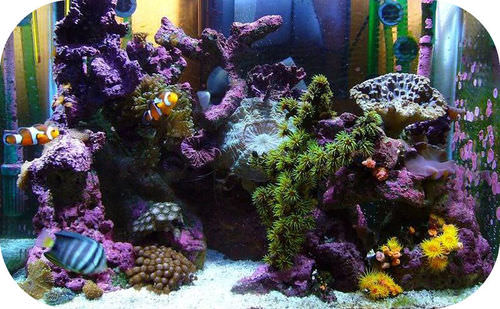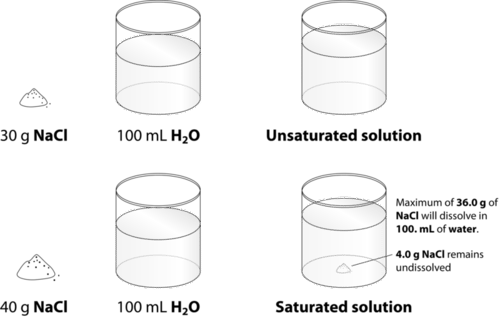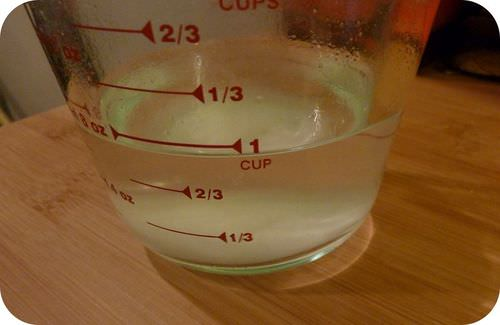# 3.2: Solubility

•• Contributed by Allison Soult
• Senior Lecturer (Chemistry) at University of Kentucky

Learning Outcomes

• List examples of solutions made from different solute-solvent combinations.
• Distinguish between saturated and unsaturated solutions.
• Explain the effects of temperature on the solubility of solids and gases.
• Use a solubility curve to determine the solubilities of substances at various temperatures.
• Explain the effect of pressure on the solubility of gases.

Previously, we looked at the primary characteristics of a solution and how water is able to dissolve solid solutes. There are many examples of solutions that do not involve water at all, or solutions that involve solutes that are not solids. The table below summarizes the possible combinations of solute-solvent states, along with examples of each.

Table $$\PageIndex{1}$$: Solute-Solvent Combinations
Solute State Solvent State Example
liquid gas water in air
gas gas oxygen in nitrogen (gas mixture)
solid liquid salt in water
liquid liquid alcohol in water
gas liquid carbon dioxide in water
solid solid zinc in copper (brass alloy)
liquid solid mercury in silver and tin (dental amalgam)

Air is a homogeneous mixture of many different gases and, therefore, qualifies as a solution. Solid-solid solutions, such as brass, bronze, and sterling silver, are called alloys. Fish depend on oxygen gas that is dissolved in the water found in oceans, lakes and rivers (see figure below). While solid-liquid and aqueous solutions comprise the majority of solutions encountered in the chemistry laboratory, it is important to be aware of the other possibilities.Figure $$\PageIndex{1}$$: Large aquariums like this salt-water tank have air continually bubbled into the water so that the fish have enough dissolved oxygen to breathe.

## Rate of Dissolving

We know that the dissolving of a solid by water depends upon the collisions that occur between the solvent molecules and the particles in the solid crystal. Anything that can be done to increase the frequency of those collisions and/or to give those collisions more energy will increase the rate of dissolving. Imagine that you were trying to dissolve some sugar in a glassful of tea. A packet of granulated sugar would dissolve faster than a cube of sugar. The rate of dissolving would be increased by stirring, or agitating the solution. Finally, the sugar would dissolve faster in hot tea than it would in cold tea.

### Surface Area

The rate at which a solute dissolves depends upon the size of the solute particles. Dissolving is a surface phenomenon, since it depends on solvent molecules colliding with the outer surface of the solute. A given quantity of solute dissolves faster when it is ground into small particles than if it is in the form of a large chunk, because more surface area is exposed. The packet of granulated sugar exposes far more surface area to the solvent and dissolves more quickly than the sugar cube.

### Agitation of the Solution

Dissolving sugar in water will occur more quickly if the water is stirred. The stirring allows fresh solvent molecules to continually be in contact with the solute. If it is not stirred, then the water right at the surface of the solute becomes saturated with dissolved sugar molecules, meaning that it is more difficult for additional solute to dissolve. The sugar cube would eventually dissolve because random motions of the water molecules would bring enough fresh solvent into contact with the sugar, but the process would take much longer. It is important to realize that neither stirring nor breaking up a solute affect the overall amount of solute that dissolves. It only affects the rate of dissolving.

### Temperature

Heating up the solvent gives the molecules more kinetic energy. The more rapid motion means that the solid or liquid solvent molecules collide with the solute with greater frequency, and the collisions occur with more force. Both factors increase the rate at which the solid or liquid solute dissolves. As we will see in the next section, a temperature change not only affects the rate of dissolving, but it also affects the amount of solute that can be dissolved.

## Types of Solutions

Table salt $$\left( \ce{NaCl} \right)$$ readily dissolves in water. Suppose that you have a beaker of water to which you add some salt, stirring until it dissolves. Then you add more, and that dissolves as well. If you keep adding more and more salt, eventually you will reach a point at which no more of the salt will dissolve, no matter how long or how vigorously you stir it. Why? On the molecular level, we know that the action of the water causes the individual ions to break apart from the salt crystal and enter the solution, where they remain hydrated by water molecules. What also happens is that some of the dissolved ions collide back again with the crystal and remain there. Recrystallization is the process of dissolved solute returning to the solid state. At some point, the rate at which the solid salt is dissolving becomes equal to the rate at which the dissolved solute is recrystallizing. When that point is reached, the total amount of dissolved salt remains unchanged.

When the solution equilibrium point is reached and no more solute will dissolve, the solution is said to be saturated. A saturated solution is a solution that contains the maximum amount of solute that is capable of being dissolved. At $$20^\text{o} \text{C}$$, the maximum amount of $$\ce{NaCl}$$ that will dissolve in $$100. \: \text{g}$$ of water is $$36.0 \: \text{g}$$. If any more $$\ce{NaCl}$$ is added past that point, it will not dissolve because the solution is saturated. What if more water is added to the solution instead? Now, more $$\ce{NaCl}$$ would be capable of dissolving, since there is additional solvent present. An unsaturated solution is a solution that contains less than the maximum amount of solute that is capable of being dissolved. The figure below illustrates the above process, and shows the distinction between unsaturated and saturated.Figure $$\PageIndex{2}$$: When $$30.0 \: \text{g}$$ of $$\ce{NaCl}$$ is added to $$100 \: \text{mL}$$ of water at $$20^\text{o} \text{C}$$, it all dissolves, forming an unsaturated solution. When $$40.0 \: \text{g}$$ is added, $$36.0 \: \text{g}$$ dissolves and $$4.0 \: \text{g}$$ remains undissolved, forming a saturated solution.

How can you tell if a solution is saturated or unsaturated? If more solute is added and it does not dissolve, then the original solution was saturated. If the added solute dissolves, then the original solution was unsaturated. A solution that has extra undissolved solute at the bottom of the container, must be saturated (see figure below).Figure $$\PageIndex{3}$$: A saturated solution of salt (sodium chloride) with undissolved solute remaining at the bottom of the measuring cup.

### Solubility Values

The solubility of a substance is the amount of that substance that is required to form a saturated solution in a given amount of solvent at a specified temperature. Solubility is often measured in grams of solute per $$100 \: \text{g}$$ of solvent. The solubility of sodium chloride in water is $$36.0 \: \text{g}$$ per $$100 \: \text{g}$$ of water at $$20^\text{o} \text{C}$$. The temperature must be specified because solubility varies with temperature. For gases, the pressure must also be specified. Solubility is specific for a particular solvent. In other words, the solubility of sodium chloride would be different in another solvent. For the purposes of this text, the solubility of a substance will refer to aqueous solubility unless otherwise specified. Solubilities for different solutes have a very wide variation, as can be seen by the data presented in the table below.

Table $$\PageIndex{2}$$: Solubility of Solutes at Different Temperatures ($$\text{g}$$ solute in $$100 \: \text{g} \: \ce{H_2O}$$)
Substance $$0^\text{o} \text{C}$$ $$20^\text{o} \text{C}$$ $$40^\text{o} \text{C}$$ $$60^\text{o} \text{C}$$ $$80^\text{o} \text{C}$$ $$100^\text{o} \text{C}$$
$$\ce{AgNO_3}$$ 122 216 311 440 585 733
$$\ce{Ba(OH)_2}$$ 1.67 3.89 8.22 20.94 101.4 __
$$\ce{C_{12}H_{22}O_{11}}$$ 179 204 238 287 362 487
$$\ce{Ca(OH)_2}$$ 0.189 0.173 0.141 0.121 __ 0.07
$$\ce{KCl}$$ 28.0 34.2 40.1 45.8 51.3 56.3
$$\ce{KI}$$ 128 144 162 176 192 206
$$\ce{KNO_3}$$ 13.9 31.6 61.3 106 167 245
$$\ce{LiCl}$$ 69.2 83.5 89.8 98.4 112 128
$$\ce{NaCl}$$ 35.7 35.9 36.4 37.1 38.0 39.2
$$\ce{NaNO_3}$$ 73 87.6 102 122 148 180
$$\ce{CO_2}$$ $$\left( 1 \: \text{atm} \right)$$ 0.335 0.169 0.0973 0.058 __ __
$$\ce{O_2}$$ $$\left( 1 \: \text{atm} \right)$$ 0.00694 0.00537 0.00308 0.00227 0.00138 0.00

## Factors Affecting Solubility

The solubility of a solid or a liquid solute in a solvent is affected by the temperature, while the solubility of a gaseous solute is affected by both the temperature and the pressure of the gas. We will examine the effects of temperature and pressure separately.

### Temperature

The solubility of the majority of solid substances increases as the temperature increases. However, the effect is difficult to predict and varies widely from one solute to another. The temperature dependence of solubility can be visualized with the help of a solubility curve, which is a graph of the solubility vs. temperature. Examine the solubility curves shown (see figure below).Figure $$\PageIndex{4}$$: A solubility curve is a graph of the solubility of a substance as a function of temperature.

Notice how the temperature dependence of $$\ce{NaCl}$$ is fairly flat, meaning that an increase in temperature has relatively little effect on the solubility of $$\ce{NaCl}$$. The curve for $$\ce{KNO_3}$$, on the other hand, is very steep; an increase in temperature dramatically increases the solubility of $$\ce{KNO_3}$$.

Several substances listed on the graph - $$\ce{HCl}$$, $$\ce{NH_3}$$, and $$\ce{SO_2}$$ - have solubilities that decrease as the temperature increases. These substances are all gases over the indicated temperature range when at standard pressure. When a solvent with a gas dissolved in it is heated, the kinetic energy of both the solvent and solute increases. As the kinetic energy of the gaseous solute increases, its molecules have a greater tendency to escape the attraction of the solvent molecules and return back to the gas phase. As a result, the solubility of a gas decreases as the temperature increases. This has some profound environmental consequences. Industrial plants situated near bodies of water often use that water as a coolant, returning the warmer water back to the lake or river. This increases the overall temperature of the water, which lowers the quantity of dissolved oxygen, affecting the survival of fish and other organisms.

Solubility curves can be used to determine if a given solution is saturated or unsaturated. Suppose that $$80 \: \text{g}$$ of $$\ce{KNO_3}$$ is added to $$100 \: \text{g}$$ of water at $$30^\text{o} \text{C}$$. According to the solubility curve, approximately $$48 \: \text{g}$$ of $$\ce{KNO_3}$$ will dissolve at $$30^\text{o} \text{C}$$. This means that the solution will be saturated, since $$48 \: \text{g}$$ is less than $$80 \: \text{g}$$. We can also determine that there will be $$80 - 48 = 32 \: \text{g}$$ of undissolved $$\ce{KNO_3}$$ remaining at the bottom of the container. Now, suppose that this saturated solution is heated to $$60^\text{o} \text{C}$$. According to the curve, the solubility of $$\ce{KNO_3}$$ at $$60^\text{o} \text{C}$$ is about $$107 \ \text{g}$$ the solution is now unsaturated, since it still contains only the original $$80 \: \text{g}$$ of solute, all of which is now dissolved. Then, suppose the solution is cooled all the way down to $$0^\text{o} \text{C}$$. The solubility at $$0^\text{o} \text{C}$$ is about $$14 \: \text{g}$$, meaning that $$80 - 14 = 66 \: \text{g}$$ of the $$\ce{KNO_3}$$ will recrystallize.

Some solutes, such as sodium acetate, do not recrystallize easily. Suppose an exactly saturated solution of sodium acetate is prepared at $$50^\text{o} \text{C}$$. As it cools back to room temperature, crystals do not immediately appear in the solution, even though the solubility of sodium acetate is lower at room temperature. A supersaturated solution is a solution that contains more than the maximum amount of solute that is capable of being dissolved at a given temperature. The recrystallization of the excess dissolved solute is a supersaturated solution can be initiated by the addition of a tiny crystal of solute, called a seed crystal. The seed crystal provides a nucleation site on which the excess dissolved crystals can begin to grow. Recrystallization from a supersaturated solution is typically very fast.

### Pressure

Pressure has very little effect on the solubility of solids or liquids, but it has a significant effect on the solubility of gases. Gas solubility increases as the partial pressure of a gas above the liquid increases. Suppose a certain volume of water is in a closed container with the space above it occupied by carbon dioxide gas at standard pressure. Some of the $$\ce{CO_2}$$ molecules come into contact with the surface of the water and dissolve into the liquid. Now suppose that more $$\ce{CO_2}$$ is added to the space above the container, causing a pressure increase. More $$\ce{CO_2}$$ molecules are now in contact with the water, so more of them dissolve. Thus the solubility increases as the pressure increases. As with a solid, the $$\ce{CO_2}$$ that is undissolved reaches an equilibrium with the dissolved $$\ce{CO_2}$$, represented by the following equation.

$\ce{CO_2} \left( g \right) \rightleftharpoons \ce{CO_2} \left( aq \right)$

At equilibrium, the rate of gaseous $$\ce{CO_2}$$ dissolving is equal to the rate of dissolved $$\ce{CO_2}$$ coming out of the solution.

When carbonated beverages are packaged, they are done so under high $$\ce{CO_2}$$ pressure so that a large amount of carbon dioxide dissolves in the liquid. When the bottle is opened, equilibrium is disrupted because the $$\ce{CO_2}$$ pressure above the liquid decreases. Immediately, bubbles of $$\ce{CO_2}$$ rapidly exit the solution and escape out of the top of the open bottle. The amount of dissolved $$\ce{CO_2}$$ decreases. If the bottle is left open for an extended period of time, the beverage becomes "flat" as more and more $$\ce{CO_2}$$ comes out of the liquid.

The relationship of gas solubility to pressure is described by Henry's law, named after English chemist William Henry (1774 - 1836). Henry's law states that the solubility of a gas in a liquid is directly proportional to the partial pressure of the gas above the liquid.